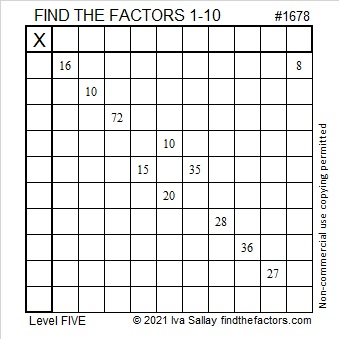# 1678 and Level 5

Contents

### Today’s Puzzle:

Using logic, write the numbers from 1 to 10 in both the first column and the top row so that this puzzle will function like a multiplication table.### Factors of 1678:

• 1678 is a composite number.
• Prime factorization: 1678 = 2 × 839.
• 1678 has no exponents greater than 1 in its prime factorization, so √1678 cannot be simplified.
• The exponents in the prime factorization are 1 and 1. Adding one to each exponent and multiplying we get (1 + 1)(1 + 1) = 2 × 2 = 4. Therefore 1678 has exactly 4 factors.
• The factors of 1678 are outlined with their factor pair partners in the graphic below.### More about the number 1678:

Because 2(839)(1) = 1678, you can calculate the only Pythagorean triple that contains the number 1678:
The smaller leg will be 2(839)(1) = 1678.
The longer leg will be 839² – 1² = 703920.
The hypotenuse will be 839² + 1² = 703922.

This site uses Akismet to reduce spam. Learn how your comment data is processed.